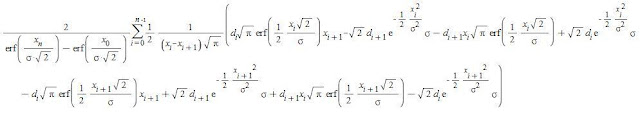## Tuesday, September 4, 2012

### A yaw-independent quantification of aerodynamic performance

The relation between yaw and aerodynamic drag makes it difficult to discern which component is really faster. Wheel manufacturers usually use sentences like "X wheel is Y seconds faster than Z wheel  over 40km" but how do they calculate that time gain if wind and bike speed is variable as well as drag as a function of yaw? The answer is using a weighting function that multiplies the drag function obtained in the wind tunnel by a factor to obtain an unique drag value.

Until now, the weighting functions that every manufacturer use haven't been known but Mavic showed his during the presentation of the Mavic CXR80. In the previous post, I worked that CDF function to obtain a normal distribution that can be used as a weighting function. Next step is to consider the drag function obtained in the wind tunnel. Typically, aerodynamic drag is considered to vary linearly between measurement points. Another aspect that has to be considered is that aparent wind speed has been variable for that weighting law but, as the fluctuations of the Reynolds numbers are small, CdA can be considered independent for a given yaw.

Let's consider a drag function with n divisions, the first abscise is called x0 and the last one, xn. The CdA for every abscise is called di, with i going from 0 to n. The weighting fuction is assumed to be a null-mean normal distribution as Mavic has showed. Now, we can define the weighed yaw-independent CdA as:erf, error function. sigma, standard deviation of the weighting function. The leading term (before the summation) takes into account that the drag function isn't defined in the whole range of possible yaw values
Let's make an example. Consider the following drag function taken from Slowtwitch's Cervélo P4 in the Tunnel article:
As you can see, the Cervélo P4 and the Trek Speed Concept are nearly tied. Considering that wind tunnel speed was set to 30mph, approximated CdA values are:

Using Mavic's weighting function with a standard deviation of 10.3388º, the terms of the summation over each interval are the following ones:

The leading term is equal to 1.056, the closer this term is to the unit, the surer we are of the conclusion. Finally, the yaw-independent CdA values of both bikes are:

The conclusion is: "A riderless Cervélo P4 "ridden" in typical conditions will produce 100*(0.003868-0.039685)/0.03868=2.598% less drag than a Trek Speed Concept with a confidence of the 100/1.056=94.697%". As the correlation between wind tunnel data and real world data has been already proved, this method, when coupled with pedaling-rider wind tunnel data, can answer the question "Which setup is fastest?" without any doubt.

That's all for today. Thanks for reading!